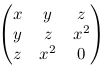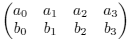Algebraic Geometry (NMAG401) - information about the course in winter semester 2018/2019.

## Basic information

The contents of the course and other basic information are available in the Student Information System.

The schedule (to be found also in the Student Information System):

• lectures on Mondays, 9.00am-10.30am in room K9,
• problem sessions (with Liran Shaul) on Wednesdays, 9.00am-10.30am in room K7.

## Credit

The credit will be granted for solved exercise problems. There will be three sets of the problems which will appear here. Requested are at least 50 % of successfully solved problems handed in withing the deadlines.

### Problem set #1 (deadline for solutions: November 19)

1. A complex algebraic subset X ⊆ A2 is called a conic if it is of the form X = V(f) where f ∈ C[x,y] is a non-zero polynomial of total degree 2. Show that every irreducible conic is isomorphic either to V(y-x2) or to V(xy-1).
2. Let C be the field of complex numbers and consider the ring R = C[x,y,z]/(xz,yz) and the element f := y-z ∈ R. Show that the localized ring Rf (the element f is made invertible) is isomorphic to the ring C[x,y±1] × C[z±1].
3. Find a birational equivalence between the complex affine line and the complex plane curve V(y3+x2-yx2) ⊆ A2. Find the rational solutions of the equation y3+x2-yx2 = 0.

### Problem set #2 (deadline for solutions: December 17)

1. Show that the image of the polynomial map f: A1 → A3 given by f(t) = (t3, t4, t5) over the field of complex numbers is a subvariety of A3. Show further that it is precisely the set of zeros of the polynomial y2 - xz and the determinant of the matrix(hint: if x is non-zero, we can put t=y/x and divide the rows of the matrix by x).

Show finally that the polynomial p = x3 - yz vanishes on the image of f and find an expression of some power pe as an element of the ideal of C[x,y,z] generated by y2 - xz and the determinant (such an expression must exist by Hilbert's Nullstellensatz).

2. Let K be an algebraically closed field. We will define a map i from the set of 2-dimesional subspaces V ⊆ K4 to the projective space P5 as follows. We arbitrarily choose two linearly independent vectors v = (a0, a1, a2, a3) and w = (b0, b1, b2, b3) in V, we write the components into a 2x4 matrixand we compute (in any fixed order) the 6 determinants d0, d1, ..., d5 of all 2x2 submatrices. Then we define i to map V to (d0 : d1 : ... : d5).

1. Show that i is a well defined injective map.
2. Show that the image X of i is a projective variety given in P5 by a single quadratic equation.

### Problem set #3 (deadline for solutions: the exam)

1. Consider over the complex numbers the subset B ⊆ A3 × P2 of all points (x,y,z, (s:t:u)) for which (s,t,u) and (x,y,z) are linearly dependent vectors.
1. Show that B is an subvariety of A3 × P2.
2. Show that the preimage of the origin 0 ∈ A3 under the projection π: B → A3 given by π(x,y,z, (s:t:u)) = (x,y,z) is isomorphic to P2, and that π restricts to an isomorphism of quasi-projective varieties B\π-1(0) → A3\{0} (i.e. B is a blow up of the origin of A3).
3. Let X=V(x2-y2-z2) ⊆ A3. Find the Zariski closure Y of π-1(X\{0}) in B and show that π restricts to an isomorphism Y\π-1(0) → X\{0}.
4. Finally show that Y has an open cover by open sets isomorphic to A2 (so we resolved the singularity of X at the origin).
2. Consider the 10-dimensional complex vector space V of all polynomials in C[x,y] of total degree at most 3, and let P9 be the corresponding projective space formed by all lines in V. Show that the irreducible cubic curves in the complex affine space A2 are parametrized by a Zariski open subset of P9 (i.e. that the irreducible cubic curves themselves form a quasi-projective variety).
3. Compute (and justify!) the Krull dimension of the Grassmann variety X from the second problem of the second problem set.

## What has been lectured

A brief overview of what has been taught in individual lectures, including references to the literature, can be found below.

October 1, 2018
Affine algebraic sets, Zariski topology, the decomposition of a noetherian topological space to irreducible components, plus the ideal of a subset of an affine space and algebraic subsets of the affine plane in the exercise session (lecture notes, sec. 1; [Ful], sec. 1.2 - 1.6, 6.1; [Ga], sec. 1.1, 1.3).
October 8, 2018
A proof for the decomposition of a noetherian topological space to irreducible components, a characterization of irreducibility via prime ideals, polynomial maps and coordinate rings, the bijection between polynomial maps and homomorphisms of coordinate rings (lecture notes, sec. 1 and 2; [Ful], sec. 1.5, 2.1 - 2.2; [Ga], sec. 1.3, 2.1).
October 15, 2018
A proof for the bijection between polynomial maps and homomorphisms of coordinate rings, polynomial isomorphisms and coordinate changes, function fields and rational functions on varieties, regular points and poles, examples (lecture notes, sec. 2; [Ful], sec. 2.2 - 2.4; [Ga], sec. 2.1, 2.3; [Sh], ch. 3).
October 22, 2018
Rational maps between varieties, the connection between rational maps with dense image and homomorphisms of function fields (lecture notes, sec. 2; [Ful], sec. 6.6; [Ga], sec. 2.1, 2.3; [Sh], ch. 3).
October 29, 2018
Birational equivalence, rational varieties, localization of rings (lecture notes, sec. 2 and 3; [Ful], sec. 6.6; [Ga], sec. 2.1, 2.3, 4.3; [Sh], ch. 3; [AM], ch. 3).
November 5, 2018
Localization at a multiplicative set, a geometric interpretation of the localization K[X] → K[X]f, radical ideals, Hilbert's Nullstellensatz (lecture notes, sec. 3; [Ful], sec. 1.7 - 1.10, 6.3; [Ga], sec. 1.2, 2.1; [AM], ch. 3).
November 12, 2018
Consequences of Hilbert's Nullstellensatz, reconstruction of an algebraic set from its coordinate ring, an open basis for the Zariski topology, local rings, in the exercise session also dicrete valuation rings (lecture notes, sec. 3 and 4; [Ful], sec. 1.7, 2.4, 2.5, 6.3; [Ga], sec. 1.2, 2.1).
November 19, 2018
Local rings at points of an algebraic set and their properties, plane curves, singular and non-singular points, tangents (lecture notes, sec. 4; [Ful], sec. 2.4, 3.1; [Ga], sec. 2.1, 4.4).
November 26, 2018
Discrete valuation rings and a characterisation of non-singular points on plane curves ([Ful], sec. 2.5, 3.2; [Ga], sec. 4.4).
December 3, 2018
Projective algebraic sets, projective Zariski topology, homogeneous ideals and homogeneous coordinate rings, decomposition to irreducible components, projective Nullstellensatz ([Ful], sec. 2.6, 4.1, 4.2; [Ga], sec. 3.1, 3.2).
December 10, 2018
Quasi-projective algebraic sets and the sheafs of regular functions on them, homomorphisms and Cartesian products of quasi-projective algebraic sets ([Ful], sec. 4.3, 6.2 - 6.4; [Ga], sec. 3.3; [Sh], sec. 5.1).
December 17, 2018
Separatedness of quasi-projective algebraic sets as a substitute for the non-Hausdorffness of the Zariski topology, projective elimination, homomorphisms from projective algebraic sets have closed image, a version of the stereographic projection in projective geometry, an exercise on blow-ups and resolution of singularities ([Ful], sec. 4.4, 6.4, 7.2; [Ga], sec. 3.3, 3.4, 4.3; [Sh], sec. 5.2).
January 7, 2019
Krull dimension, computation of the dimension for affine and projective spaces ([Ful], sec. 6.5; [Ga], sec. 1.4, 4.1 - 4.2; [Sh], sec. 5.3 - 5.4, 6.1).

## Literature

I will write and update a draft version of lecture notes during the semester: [Full text in PDF]

As far as other sources are concerned, algebraic geometry has gradually grown to a very broad field with thousands of pages written about it from several points of view. To get an impression (or to get an idea where to go next for those interested in the topic), check this blog on mathoverflow.net. The core of the lecture is presented according to the following sources available in PDF:

 [Ga] A. Gathmann, Algebraic geometry, notes from a course in Kaiserslautern, 2002/2003. [Full text in PDF] [Ful] W. Fulton, Algebraic Curves (An Introduction to Algebraic Geometry), 2008. [Full text in PDF]

The lectures may also involve facts from the following (off-line) sources:

 [AM] M. F. Atiyah, I. G. MacDonald, Introduction to Commutative Algebra, Addison-Wesley Publishing Co., 1969. [CLO] D. Cox, J. Little, D. O'Shea, Ideals, varieties, and algorithms, Second Edition, Springer, New York, 2005. [Na] M. Nagata, Local Rings, John Wiley & Sons, 1962. [Nee] A. Neeman, Algebraic and Analytic Geometry, LMS Lecture Note Series 345, Cambridge, 2007. [Sh] I. R. Shafarevich, Basic algebraic geometry 1, Varieties in projective space, 2. vyd., Springer-Verlag, Berlin, 1994.